You have no items in your shopping cart.
Search

# Set Irrigation Times

Use this form to determine the set time required to fulfill a given water application, irrigation area, and flow rate.

## Set Irrigation Times

Net Water Application:
System Efficiency:
Minimum efficiency of DuCaR Sprinklers is 80%.
%
Irrigated Area:
Flow Rate:
Set Time:

### The Equation

This is the formula this calculator uses to determine the set times for an irrigation system.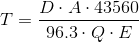Where: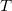= Set Time (hr)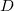= Net water application (in)= Irrigated area (acres)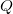= Flow Rate (gpm)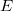= System efficiency

Reference: Washington State University